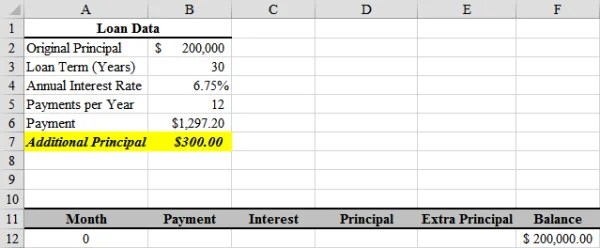# How To Calculate Number of Loan Payments Left

## Basic Information related to Calculate Number of Loan Payments LeftBorrowing money in today’s world has become easier as compared to the previous time. One of the reasons why it has become easier because of the increased tuition fee of colleges and students cant spend money on their education because it is expensive.

A new online system has been introduced in which many people can track their loan payments online and determine how much money they must pay back and how many times they need to make the payment. If your lender hasn’t provided with you a portal then don’t worry!

There is a mathematical formula which is very easy and helpful in calculating the number of loan payments that are still to be made. Further, are some steps discussed that will help you in easily calculating the number of payments that are still pending.

## Step by step guideline to Calculate Number of Loan Payments LeftHere are the steps that will help you in calculating the number of loan payments that are left, if you don’t have a portal:

• You have to determine the amount that is remaining of principal, payments and the interest rate on the loan. For example, a former student has \$20,000 remaining in principal on a college loan with 6 percent interest, and each month he pays \$300 on the loan. Translate the interest rate to the interest rate per month by dividing 6 percent by 12, which equals 0.005.
• Now, you have to divide the principal of the loan by the payment amount. In our example, \$20,000 divided by \$300 equals 66.6667. Then multiply that number by the interest rate per month. In our example, 66.6667 times 0.005 equals 0.3333.
• Further, you have to subtract from 1 the number calculated in Step 2. In the example, 1 minus 0.3333 equals 0.6667.

• Add 1 to the interest rate per month. In our example, 1 plus 0.005 equals 1.005.

• Calculate the negative logarithm of the number calculated in Step 3. Use a calculator with a logarithm function (see Resources). Plug in 0.6667 and hit the “log” key. In our example, -log(0.6667) equals 0.176070.

• Calculate the log of the number calculated in Step 4. In our example, log(1.005) equals 0.002166.

Divided the number calculated in Step 5 by the number calculated in Step 6 to determine the number of remaining payments. In our example, 0.176070 divided by 0.002166 equals 81.29. So the former student will have 81 remaining payments of \$300 and one payment of \$87 (.29 times \$300), for a total of \$24,387 (principal plus interest).